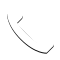Select Page

In this post, I will be sharing with you about this alternative mortgage called Price Level Adjusted Mortgage (PLAM). This mortgage is one of the many mortgages that is used such as the Fixed Rate Mortgage (FRM) and Adjustable Rate Mortgage (ARM). I will explain to you about the way PLAM works. It may or may not be applicable to your country. Hardly applicable for Singapore residential mortgage.

### PLAM Factors

PLAM makes use of the Real Interest Rate for your loan. Real Interest Rate = Nominal Rate – Inflation Rate. The elements for PLAM includes:

• The contract rate which is = Real Interest Rate
• Inflation is added to the remaining loan balance on an ex-post basis.

Ex-post basis means that the inflation added is the inflation that has been recorded in this ending year. It is not the inflation that you forecast in the following year.

Since the monthly loan payment does not include inflation rate it could be low and solve affordability problems. The inflation will be added at the end of the year which would also reduce the interest rate risk problem for the lenders.

### PLAM Calculation

The way to do a PLAM calculation is in this steps. The idea is to treat every year as though you have taken a new loan yearly. Things to note, every year you will have a new loan balance and your number of period decreases.

1. Find out the monthly payment for the Year 1.
2. Find out the remaining loan balance after Year 1.
3. Add the inflation rate to the remaining loan balance to find out the remaining loan balance for Year 2.
4. Repeat steps 1 to 3 for the monthly payment for year 2.

Borrow \$100,000 for 30 years, monthly payments. Current Real Rate = 6% with Annual Payment Adjustments. Assumptions of inflation rate for calculation.

 End of Year Inflation 1 4% 2 -3% 3 2%
##### Year 1 Payments

Simply use a financial calculator to calculate like an FRM

1. PV = \$100,000
2. N = 360
3. i/y = 6%
4. FV = 0
5. CPT PMT = -\$599.55 (Monthly Payment for year 1)
##### Year 2 Payments

Remember that you need to add inflation to the remaining balance from year 1. Treat this as a new loan.

Remaining balance at end of year 1: Use AMORT function, P1=1 P2=12 BAL=\$98,771.99

Inflation adjustment: \$98,771.99(1.04) = \$102,722.87 (Balance left to be brought over to year 2)

Treating as a new loan to calculate monthly payment:

1. PV = \$102,722.87
2. N = 348
3. i/y = 6%
4. FV = 0
5. CPT PMT = -\$623.53 (Monthly Payment for year 2)
##### Year 3 Payments

Remaining balance at end of year 2: Use AMORT function, P1=1 P2=12 BAL=\$101,366.97

Inflation adjustment: \$101,366.97(0.97) = \$98325.95 (Balance left to be brought over to year 3)

Treating as a new loan to calculate monthly payment:

1. PV = \$98325.95
2. N = 336
3. i/y = 6%
4. FV = 0
5. CPT PMT = -\$604.83 (Monthly Payment for year 3)

### Closing The Loan At Year 3

You will still need to calculate the remaining loan balance by finding the loan balance after end of year 3 while adding the adjustment for inflation to find out loan amount repayable. By using the cashflow method to calculate the Internal Rate of Return(IRR) it will amount to around 6.97% annual effective borrowing cost.

### Problems Associated With PLAM

1. If you have noticed in year 2, you had a negative amortization due to inflation. There is a possibility that loan balance increases faster than property value and thus more incentive to default.
2. The increase in inflation may not mean an increase in income. Thus, if inflation rises more than income, there is a possibility of default.
3. Lenders are not able to forecast inflation and lag adjustments. They use last year’s inflation for next year’s payment. This means that lenders can only adjust the rate after 1 year.

## Get In Touch

3 + 7 =Click To Call